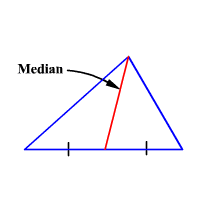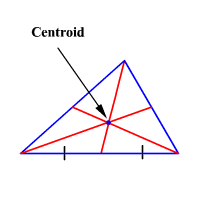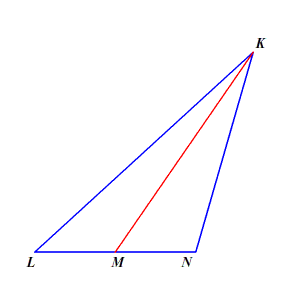# Medians of a Triangle

A median of a triangle is a line segment drawn from a vertex to the midpoint of the opposite side of the vertex.The medians of a triangle are concurrent at a point. The point of concurrency is called the centroid.Example:

In the figure shown, $LN=14$ units, $NK=22$ units, and $KL=34$ units. If $\stackrel{¯}{KM}$ is a median of the triangle, find the length of $\stackrel{¯}{LM}$ .The fact that $\stackrel{¯}{KM}$ is a median tells us that $M$ must be a midpoint of $\stackrel{¯}{LN}$ . So, to find $LM$ , all we need to do is divide $LN$ by $2$ .

Note that this problem gives us a couple of pieces of irrelevant information. We don't need to know the values of $NK$ and $KL$ .